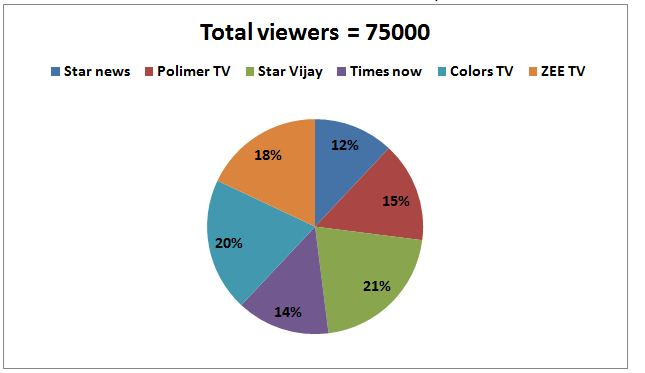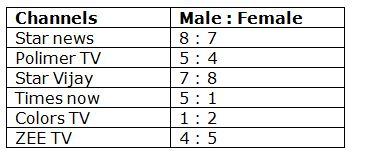# SBI Clerk Prelims 2020 Quantitative Aptitude Questions (Day-08)

Dear Aspirants, Our IBPS Guide team is providing new series of Quantitative Aptitude Questions for SBI Clerk Prelims 2020 so the aspirants can practice it on a daily basis. These questions are framed by our skilled experts after understanding your needs thoroughly. Aspirants can practice these new series questions daily to familiarize with the exact exam pattern and make your preparation effective.

[WpProQuiz 7630]

Ensure Your Ability Before the Exam – Take SBI Clerk 2020 Prelims Free Mock Test

Inequality

Directions (Q. 1 – 5): Each question contains a statement followed by Quantity I and Quantity II. Read the contents clearly and give answer as,

a) Quantity I > Quantity II

b) Quantity I ≥ Quantity II

c) Quantity II > Quantity I

d) Quantity II ≥ Quantity I

e) Quantity I = Quantity II or Relation cannot be established

1) Quantity I: The present age of Asha is 12 years more the present age of Rahul. After 5 years the ratio of ages of Rahul and Asha will be 3: 5, then find the age of Rahul, after 2 years?

Quantity II: The present age of Naveen is twice the present age of Priya. The ratio between the present ages of Gowrish and Priya is 7: 4. If the age of Naveen after 6 years will be 30 years, then find the age of Gowrish before 6 years?

2) A started the business investing Rs. 19000 and after 6 months B joined him with Rs. 28000. At the end of one year the total profit is Rs.16500.

Quantity I: A’s profit share?

Quantity II: B’s profit share?

3) Quantity I: Two trains running the same direction at the speed of 60kmph and 80kmph respectively. If the length of the trains is 200m and 100m respectively, then find the time taken by first train crosses the second train?

Quantity II: If the train A crosses the pole in 18 seconds at the speed of 60kmph and the same train crosses the 700m long train which is running opposite direction of train A at the speed of 20kmph, then find the time taken by train A crosses the another train?

4) Quantity I: Time taken by P to complete 1/5th of work, if Q takes 6 days to complete 3/5th of work and together they take 5 days to complete 3/4th of work?

Quantity II:  Time taken by a train to cross a platform of length 60 km given that it crosses a pole in two and a half hours running at 60 km/hr?

5) A box contains 4 Red balls, 3 white balls, 5 orange balls and 6 blue balls.

Quantity I: If two balls are drawn out randomly, then find the probability that both the balls are either orange or blue?

Quantity II: If four balls are drawn out randomly, then find the probability that all the balls are of different colours?

Data Interpretation

Directions (Q. 6 – 10): Study the following information carefully and answer the given questions?

The following pie chart shows the percentage distribution of total number of television viewers of different channels in a certain cityThe following table shows the ratio of male and female television viewers.6) Find the difference between the total number of male television viewers of Star news to that of total number of female television viewers of Star Vijay?

a) 2400

b) 2800

c) 3600

d) 3200

e) None of these

7) Find the ratio between the total number of television viewers of Polimer TV to that of total number of female television viewers of Colors TV?

a) 9 : 8

b) 5 : 3

c) 11 : 7

d) 23 : 15

e) None of these

8) Total number of television viewers of Times Now is what percentage of total number of male television viewers of ZEE TV?

a) 215 %

b) 160 %

c) 140 %

d) 175 %

e) None of these

9) Find the average number of male television viewers of Polimer TV, Star Vijay and Colors TV together?

a) 5800

b) 6200

c) 6350

d) 6700

e) None of these

10) Total number of female television viewers of Star news is what percentage more/less than the total number of male television viewers of ZEE TV?

a) 10 % more

b) 20 % less

c) 30 % less

d) 25 % more

e) None of these

Directions (1-5):

Quantity I:

Present age of Asha = 12 + Present age of Rahul

After 10 years, the ratio of ages of Rahul and Asha = 3: 5 (3x, 5x)

5x – 3x = 12

2x = 12

x = 6

The age of Rahul, after 2 years = 3x – 5 + 2 = 15 years

Quantity II:

The ratio of present age of Naveen and Priya = 2 : 1

The ratio of present age of Gowrish and Priya = 7 : 4

The present age of Naveen, Priya and Gowrish = 8 : 4 : 7

The age of Naveen, after 6 years = 30 years

The present age of Naveen = 24 years

8’s = 24

1’s = 3

The age of Gowrish before 6 years = 7x – 6 = 15 years

Quantity I = Quantity II

Profit share=19000 * 12 : 28000 * 6 =19:14

Quantity I,

A’s share

= > 19/33*16500

= > Rs.9500

Quantity II,

B’s share

= > 14/33 * 16500

= > 7000

Quantity I > Quantity II

Quantity I,

300 = (80-60) * (5/18) * x

300 = 20 * (5/18) * x

x = 54 seconds

Quantity II,

x = 60 * 5/18 * 18

x = 300m

300+700 = 80 * 5/18 * x

x = 45 seconds

Quantity I > Quantity II

Quantity I:

P and Q completes 3/4th work in 5 days, so complete 1 work in 4/3 * 5 = 20/3 days

Q complete 3/5 work in 6 days, so complete work in 5/3 * 6 = 10 days

So in 1 day P completes = 3/20 – 1/10 = 1/20

So to complete 1/5th work = 1/5 * 20 = 4 days

Quantity II:

Length of train = 2.5 * 60 = 150 km

So time taken to cross platform of length 60 km with speed 60 km/hr

= (150+60)/60

= 3.5 hrs

Hence, Quantity I > Quantity II

Quantity I:

Total number of balls = 4 + 3 + 5 + 6 = 18 balls

n(S) = 18C2

Probability that both the balls are either Orange or blue

n(E) = 5C2 or 6C2

P(E) = n(E) / n(S) = [5C2 or 6C2] / 18C2

P(E) = 25 / 153

Quantity II:

n(S) = 18C4

Probability that all the balls are of different colours

n(E) = 4C1 and 3C1 and 5C1 and 6C1

P(E) = n(E) / n(S) = [4C1 or 3C1 or 5C1 or 6C1] / 18C4

P(E) = (4 * 3 * 5 * 6) / [(18 * 17 * 16 * 15) / (1 * 2 * 3 * 4)]

P(E) =  2 / 17

Quantity I > Quantity II

Directions (6-10):

The total number of male television viewers of Star news

= > 75000*(12/100)*(8/15) = 4800

The total number of female television viewers of Star Vijay

= > 75000*(21/100)*(8/15) = 8400

Required difference = 8400 – 4800 = 3600

The total number of television viewers of Polimer TV

= > 75000*(15/100) = 11250

The total number of female television viewers of Colors TV

= > 75000*(20/100)*(2/3) = 10000

Required ratio = 11250 : 10000 = 9 : 8

Total number of television viewers of Times Now

= > 75000*(14/100) = 10500

Total number of male television viewers of ZEE TV

= > 75000*(18/100)*(4/9) = 6000

Required % = (10500/6000)*100 = 175 %

The total number of male television viewers of Polimer TV, Star Vijay and Colors TV together

= > 75000*(15/100)*(5/9) + 75000*(21/100)*(7/15) + 75000*(20/100)*(1/3)

= > 6250 + 7350 + 5000 = 18600

Required average = 18600/3 = 6200

Total number of female television viewers of Star news

= > 75000*(12/100)*(7/15) = 4200

Total number of male television viewers of ZEE TV

= > 75000*(18/100)*(4/9) = 6000

Required % = [(6000 – 4200)/6000]*100 = 30 % less

 Check Here to View SBI Clerk Prelims 2020 Quantitative Aptitude Questions Day 07 Day 06 Day 05 Click Here for SBI Clerk 2020 – Detailed Exam Notification